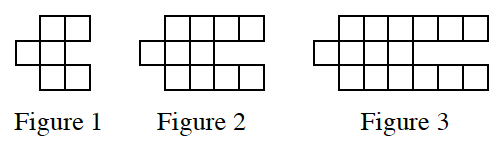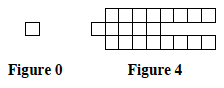Home > CC3 > Chapter 6 > Lesson 6.1.2 > Problem6-16

6-16.

Examine the tile pattern shown below. 6-16 HW eTool (CPM). Homework Help ✎1. On graph paper, draw Figure 0 and Figure 4.

How many tiles does the pattern grow by in each figure?2. How many tiles will Figure 10 have? Justify your answer.

Find the equation for the pattern.

Use the eTool below to examine the pattern and draw Figures 0 and 4.
Click the link at right for the full version of the eTool: CC3 6-16 HW eTool